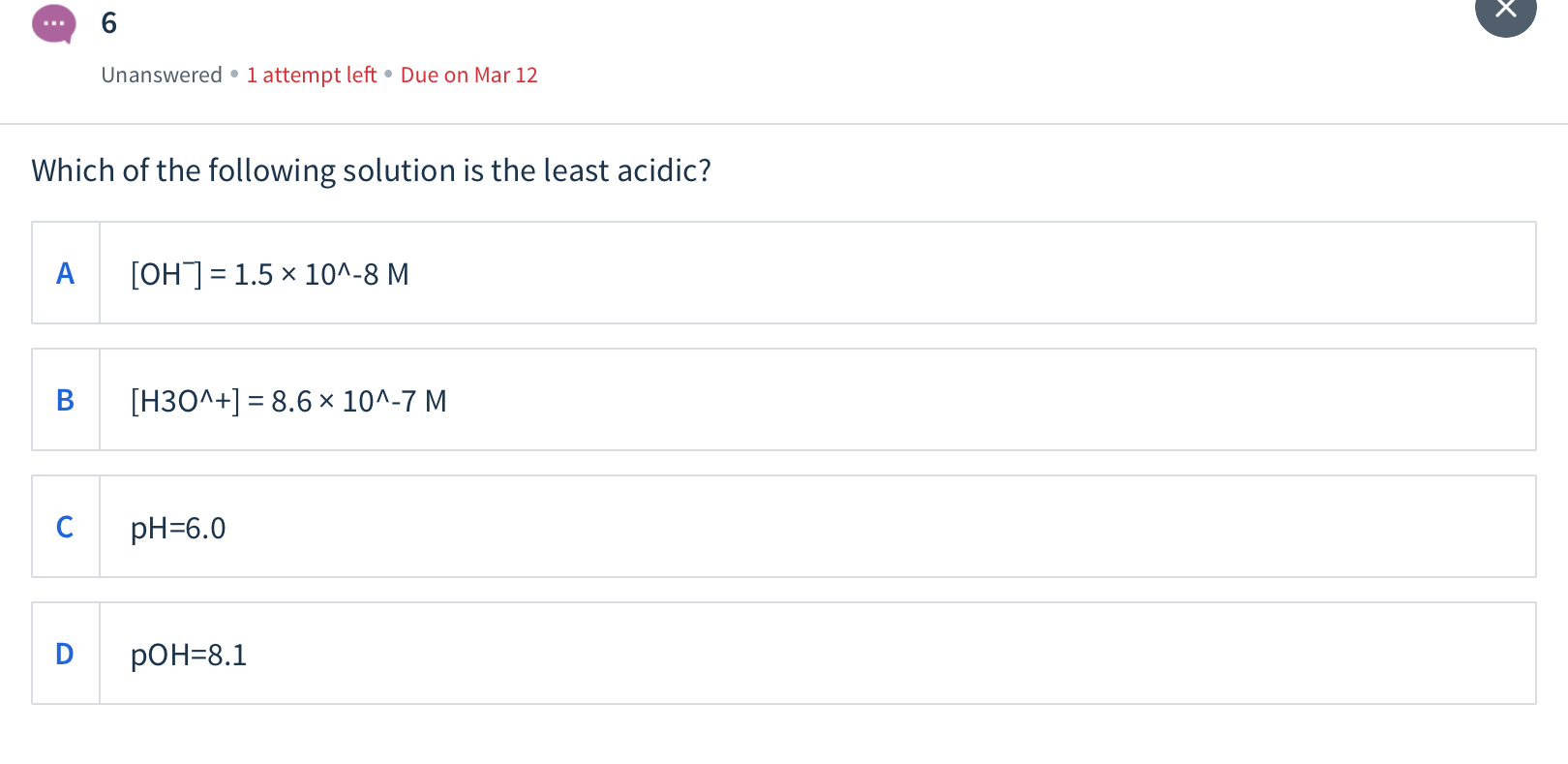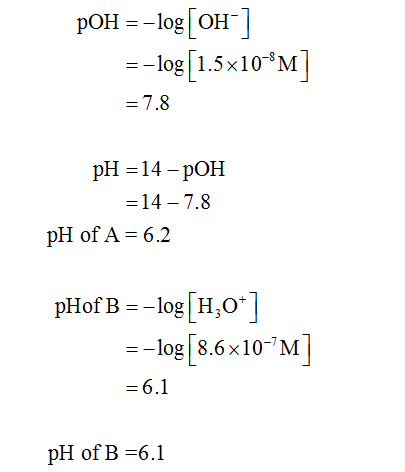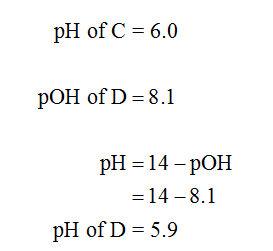# Unanswered • 1 attempt left • Due on Mar 12Which of the following solution is the least acidic?[OH]= 1.5 x 10^-8 MB[H3O^+] = 8.6 x 10^-7 MpH=6.0pOH=8.1

Question
1 viewshelp_outlineImage TranscriptioncloseUnanswered • 1 attempt left • Due on Mar 12 Which of the following solution is the least acidic? [OH]= 1.5 x 10^-8 M B [H3O^+] = 8.6 x 10^-7 M pH=6.0 pOH=8.1 fullscreen
check_circle

Step 1

p H of solution A and B can be calculated using the equation given below:Step 2

p H of solution C and D can be calculated using the equation given below:...

### Want to see the full answer?

See Solution

#### Want to see this answer and more?

Solutions are written by subject experts who are available 24/7. Questions are typically answered within 1 hour.*

See Solution
*Response times may vary by subject and question.
Tagged in